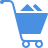Cart (0)

A dependent variable that is lagged in time. For example, if Yt is the dependent variable, then Yt-1 will be a lagged dependent variable with a lag of one period. Lagged values are used in Dynamic Regression modeling. They are also used in ARIMA modeling where it is assumed that the forecast of the next period depends on past values of the same series.

Previous Entry
Next Entry